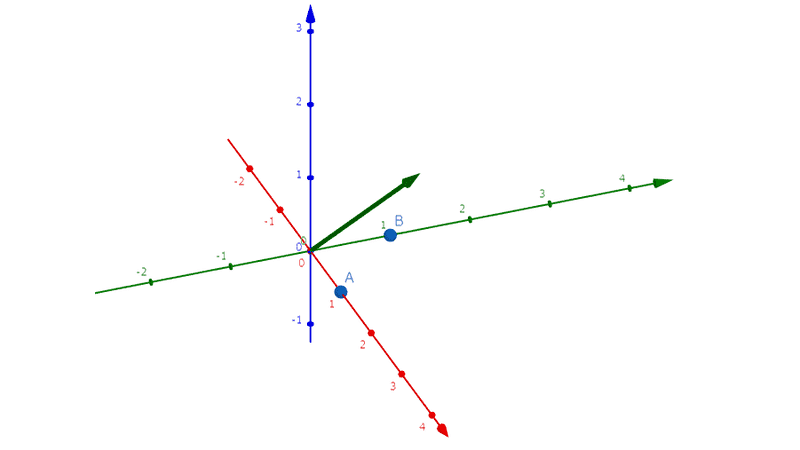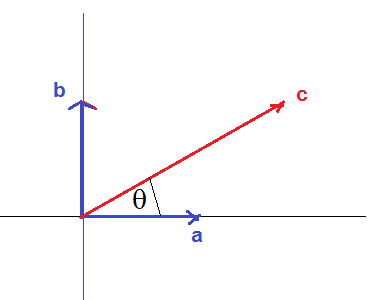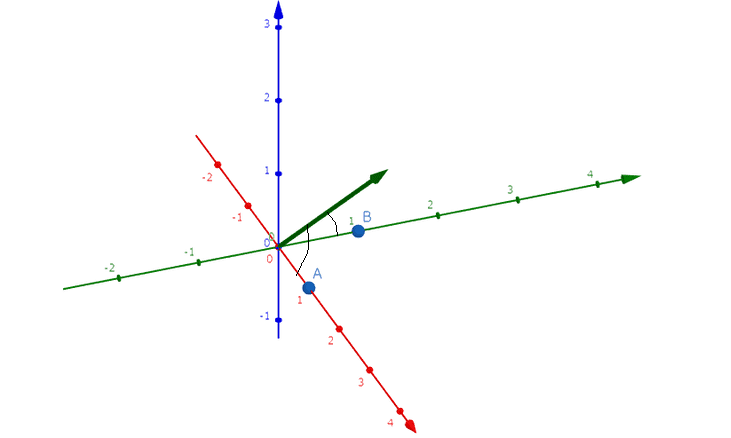# Dependence condition

## Homework Statement

>There are three vector $$\vec a ,\vec b, \vec c$$ in three-dimensional real vector space, and the inner product between them $$\vec a . \vec a=\vec b.\vec b=\vec a.\vec c=1, \vec a.\vec b=0, \vec c.\vec c=4$$ When setting $$x = \vec b.\vec c$$ ,
(dot here means dot product)
answer the following question: when $$\vec a ,\vec b, \vec c$$ are linearly dependent, find all possible values of $$x$$

## Homework Equations

3. The Attempt at a Solution

For dependent condition

\begin{align} (a×b)·c &= 0\\ a·(b×c) &= 0\\ a(bc \sin θ)&=0 \end{align}

So ## \theta= 0## and ##\pi##

Then
\begin{align} x&=|b||c| \cos \theta \\ x&=2 \cos \theta \\ \implies x &= 2 \cos 0, x = 2 \cos \pi \\ x &= \mp 2 \end{align}

Am I right?

Last edited:

You haven't said what you mean by ##\theta## and your equation ##a(bc (sin(\theta))=0## looks suspect to me. Where do you take into account the angle between ##\vec b \times \vec c## and ##\vec a##?

You haven't said what you mean by ##\theta## and your equation ##a(bc (sin(\theta))=0## looks suspect to me. Where do you take into account the angle between ##\vec b \times \vec c## and ##\vec a##?
$$\theta$$ is angle?
\begin{align}
(a×b)·c &= 0\\
a·(b×c) &= 0\\
a(bc \sin θ)&=0
\end{align}

i think this is cross product? i tried to apply scalar triple product like the one when you find area of parallelogram ? but i wasnt sure

Ray Vickson
Homework Helper
Dearly Missed

## Homework Statement

>There are three vector $$\vec a ,\vec b, \vec c$$ in three-dimensional real vector space, and the inner product between them $$\vec a . \vec a=\vec b.\vec b=\vec a.\vec c=1, \vec a.\vec b=0, \vec c.\vec c=4$$ When setting $$\vec x = \vec b.\vec c$$ ,
(dot here means dot product)
answer the following question: when $$\vec a ,\vec b, \vec c$$ are linearly dependent, find all possible values of $$\vec x$$

## The Attempt at a Solution

For dependent condition

\begin{align} (a×b)·c &= 0\\ a·(b×c) &= 0\\ a(bc \sin θ)&=0 \end{align}

So ## \theta= 0## and ##\pi##

Then
\begin{align} x&=|b||c| \cos \theta \\ x&=2 \cos \theta \\ \implies x &= 2 \cos 0, x = 2 \cos \pi \\ x &= \mp 2 \end{align}

Am I right?[/B]

(1) Remove the bold font; it looks like you are yelling.
(2) Remove the arrow above ##x##, because ##x## is not a vector---it is a scalar.

(1) Remove the bold font; it looks like you are yelling.
(2) Remove the arrow above ##x##, because ##x## is not a vector---it is a scalar.
ok, done

Dick
Homework Helper
ok, done

There's no cross product in your given relations. You know ##|a|=1## and rotating all of your vectors by the same amount doesn't change anything. So why not set ##\vec a=(1,0,0)## and see what the relations are telling you in terms of coordinates?

There's no cross product in your given relations. You know ##|a|=1## and rotating all of your vectors by the same amount doesn't change anything. So why not set ##\vec a=(1,0,0)## and see what the relations are telling you in terms of coordinates?
thanks! but why set ##\vec a=(1,0,0)## and set to what? i though i can use cross product to test dependency?

Dick
Homework Helper
thanks! but why set ##\vec a=(1,0,0)## and set to what? i though i can use cross product to test dependency?

The question isn't asking you about dependency, it's asking for a range of values. And like I said, the point to setting ##\vec a=(1,0,0)## is just for definiteness. You could set it to any vector with norm 1. Then put ##\vec b=(b_x, b_y, b_z)## and ##\vec c=(c_x, c_y, c_z)## and see what the relations say in terms of those coordinates.

The question isn't asking you about dependency, it's asking for a range of values. And like I said, the point to setting ##\vec a=(1,0,0)## is just for definiteness. You could set it to any vector with norm 1. Then put ##\vec b=(b_x, b_y, b_z)## and ##\vec c=(c_x, c_y, c_z)## and see what the relations say in terms of those coordinates.

do you mean i can pick any vector that match the inner product?
##\vec a=(1, 0, 0)## , ##\vec b=(0, 1, 0)## (?)
is it possible when ##a.c cos \theta= 1##
##1.2 cos \theta=1 ## and find the ##\theta##?

Dick
Homework Helper
do you mean i can pick any vector that match the inner product?
##\vec a=(1, 0, 0)## , ##\vec b=(0, 1, 0)## (?)
is it possible when ##a.c cos \theta= 1##
##1.2 cos \theta=1 ## and find the ##\theta##?

The picture you should have in your head is taking the three vectors and rotating them all together. And yes, since ##\vec a## and ##\vec b## are unit vectors and orthogonal you could choose them that way. But now you can't fix ##\vec c## since it can have a variety of positions with respect to the other two. Just see what the dot product relations tell you about the coordinates of ##\vec c##.

The picture you should have in your head is taking the three vectors and rotating them all together. And yes, since ##\vec a## and ##\vec b## are unit vectors and orthogonal you could choose them that way. But now you can't fix ##\vec c## since it can have a variety of positions with respect to the other two. Just see what the dot product relations tell you about the coordinates of ##\vec c##.
does this mean that a and b and c is in the same plane? and since a and b are independent to each other, it span the plane? and c is inside it?
so i can write c as linear combination of a and b so c= ka+sb?

Dick
Homework Helper
does this mean that a and b and c is in the same plane? and since a and b are independent to each other, it span the plane? and c is inside it?
so i can write c as linear combination of a and b so c= ka+sb?

I don't see anything in the problem that would indicate the vectors are coplanar. Why do you think that?

I don't see anything in the problem that would indicate the vectors are coplanar. Why do you think that?
it is said that a.b=0 ?
if ##\vec a=(1, 0, 0)## and ##\vec b=(0, 1, 0)## ,it is independent to each other? so c is in the span of two of them since three of them are dependent? and they are on the same plane?

Dick
Homework Helper
it is said that a.b=0 ?
if ##\vec a=(1, 0, 0)## and ##\vec b=(0, 1, 0)## ,it is independent to each other? so c is in the span of two of them since three of them are dependent? and they are on the same plane?

You're told they are three dimensional vectors. Two independent vectors don't span the space. Nothing in the problem indicates the three are dependent.

You're told they are three dimensional vectors. Two independent vectors don't span the space. Nothing in the problem indicates the three are dependent.
it is said that when a, b, c are linearly dependent find possible value of x ?
You're told they are three dimensional vectors. Two independent vectors don't span the space. Nothing in the problem indicates the three are dependent.
it is said in the problem a,b,c are linear dependence
##1.2 cos \theta=1## ,from here the angle between a and c is 60 degree? since a and b is orthogonal to each other b and c will be 30 degree? am i right?

Dick
Homework Helper
it is said that when a, b, c are linearly dependent find possible value of x ?

it is said in the problem a,b,c are linear dependence
##1.2 cos \theta=1## ,from here the angle between a and c is 60 degree? since a and b is orthogonal to each other b and c will be 30 degree? am i right?

All right. Sorry, now I see that it does say they are linearly dependent. So almost true. There's another choice of angle that works as well.

All right. Sorry, now I see that it does say they are linearly dependent. So almost true. There's another choice of angle that works as well.

can i use the fact that a and b span the plane and c is inside the plane? so i can express c as linear combination of them altough it is in 3 dimentional?
x=b.c cos theta since theta is 30 , ##x=b.c cos 30 = \sqrt 3## ?

Dick
Homework Helper
can i use the fact that a and b span the plane and c is inside the plane? so i can express c as linear combination of them altough it is in 3 dimentional?
x=b.c cos theta since theta is 30 , ##x=b.c cos 30 = \sqrt 3## ?

That's one possibility. There's another. There are two different positions in the plane where there's a 60 degree angle between ##\vec a## and ##\vec c##. Draw a picture.

That's one possibility. There's another. There are two different positions in the plane where there's a 60 degree angle between ##\vec a## and ##\vec c##. Draw a picture.

##\vec a=(1,0,0)## ##\vec b=(0,1,0)## ##\vec c=(1,1,0)## , i choose this point . and clearly c is in span of a and b, can you give me more hint?

ehild
Homework Helper
What is the magnitude of ##\vec c##? What can be the components then?

What is the magnitude of ##\vec c##? What can be the components then?
i was wrong , ##\vec c=(1,1,\sqrt2)##

That's one possibility. There's another. There are two different positions in the plane where there's a 60 degree angle between ##\vec a## and ##\vec c##. Draw a picture.
sorry i was wrong let ##\vec c=(1,1,\sqrt2)##

What is the magnitude of c##? What can be the components then?
##\veccan you point how can i see the angle here?

#### Attachments

ehild
Homework Helper
sorry i was wrong let ##\vec c=(1,1,\sqrt2)##
Are the vectors ## \vec a (1,0,0)##, ## \vec b (0,1,0)##, ## \vec c (1,1,√2)## dependent?

Are the vectors ## \vec a (1,0,0)##, ## \vec b (0,1,0)##, ## \vec c (1,1,√2)## dependent?
a and b independent but c is dependent to a and b ?

ehild
Homework Helper
a and b independent but c is dependent to a and b ?
Two vectors of the three are independent, and the third can be combined from the two others. You can write vector c as linear combination of a and b vectors, and find the coefficients from the given data.

Two vectors of the three are independent, and the third can be combined from the two others. You can write vector c as linear combination of a and b vectors, and find the coefficients from the given data.
yes but is the picture that i drew right? how can i find the angle between them?

##\vecView attachment 226262

ehild
Homework Helper
You define angles between two vectors, a and c, b and c. But you draw the vector c out of the plane spread by a and b, as if it had a component perpendicular to both a and b, so independent from them. Remember, they are dependent in 3 dimension. That means they are in one plane.#### Attachments

ehild
Homework Helper
##\vecView attachment 226262
can you point how can i see the angle here?
There are two angles here, between a and c and between b and c. Are the vectors in the drawing dependent?#### Attachments

You define angles between two vectors, a and c, b and c. But you draw the vector c out of the plane spread by a and b, as if it had a component perpendicular to both a and b, so independent from them. Remember, they are dependent in 3 dimension. That means they are in one plane.

View attachment 226279
yeah in my picture i chose ##c=(1,1, \sqrt2)## , is it wrong?
There are two angles here, between a and c and between b and c. Are the vectors in the drawing dependent?
View attachment 226280

i think here i was wrong, the three vector are independent to each other in my drawing because they are not in same plane right?
so what coordinate should i choose for c? is ##c=(1,1, \sqrt2)## wrong?

You define angles between two vectors, a and c, b and c. But you draw the vector c out of the plane spread by a and b, as if it had a component perpendicular to both a and b, so independent from them. Remember, they are dependent in 3 dimension. That means they are in one plane.

View attachment 226279
how can i draw like you drew c is in the span of a and b? what coordinate should i choose?

There are two angles here, between a and c and between b and c. Are the vectors in the drawing dependent?
View attachment 226280

i think here i was wrong, the three vector are independent to each other in my drawing because they are not in same plane right?
so what coordinate should i choose for c? is ##c=(1,1, \sqrt2)## wrong?

ehild
Homework Helper
yeah in my picture i chose ##c=(1,1, \sqrt2)## , is it wrong?
Is ##\vec c=(1,1, \sqrt2)## dependent of (1,0,0) and (0,0,1)? How can you decide if three vectors are independent or dependent?

i think here i was wrong, the three vector are independent to each other in my drawing because they are not in same plane right?
so what coordinate should i choose for c? is ##c=(1,1, \sqrt2)## wrong?
If the plane is spread by a and b, what is the third coordinate of all vectors in that plane?

ehild
Homework Helper
i think here i was wrong, the three vector are independent to each other in my drawing because they are not in same plane right?
so what coordinate should i choose for c? is ##c=(1,1, \sqrt2)## wrong?
It is wrong, of course.
If a=(1,0,0) and b=(0,1,0), what should be the third coordinate of c so it is a linear combination of a and b?
The third coordinate is a linear combination of the third coordinates of both a and b, two zeros. What do you get if you combine two zeros?

Is ##\vec c=(1,1, \sqrt2)## dependent of (1,0,0) and (0,0,1)? How can you decide if three vectors are independent or dependent?

the determinant is -1 so it is independent to each other?

If the plane is spread by a and b, what is the third coordinate of all vectors in that plane?

if the plane is spread by a and b, the third coordinate is linear combination of a and b